Test: Dimensional Geometry - 1

# Test: Dimensional Geometry - 1

Test Description

## 20 Questions MCQ Test Topic-wise Tests & Solved Examples for IIT JAM Mathematics | Test: Dimensional Geometry - 1

Test: Dimensional Geometry - 1 for Mathematics 2023 is part of Topic-wise Tests & Solved Examples for IIT JAM Mathematics preparation. The Test: Dimensional Geometry - 1 questions and answers have been prepared according to the Mathematics exam syllabus.The Test: Dimensional Geometry - 1 MCQs are made for Mathematics 2023 Exam. Find important definitions, questions, notes, meanings, examples, exercises, MCQs and online tests for Test: Dimensional Geometry - 1 below.
Solutions of Test: Dimensional Geometry - 1 questions in English are available as part of our Topic-wise Tests & Solved Examples for IIT JAM Mathematics for Mathematics & Test: Dimensional Geometry - 1 solutions in Hindi for Topic-wise Tests & Solved Examples for IIT JAM Mathematics course. Download more important topics, notes, lectures and mock test series for Mathematics Exam by signing up for free. Attempt Test: Dimensional Geometry - 1 | 20 questions in 60 minutes | Mock test for Mathematics preparation | Free important questions MCQ to study Topic-wise Tests & Solved Examples for IIT JAM Mathematics for Mathematics Exam | Download free PDF with solutions
 1 Crore+ students have signed up on EduRev. Have you?
Test: Dimensional Geometry - 1 - Question 1

### Let the coordinate axes be inclined at an angle θ. Then the distance between two points P(x1, y1) and Q(x2, y2) is given by

Detailed Solution for Test: Dimensional Geometry - 1 - Question 1

Note that if the axes are inclined at 90° , then the distance is given by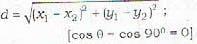Test: Dimensional Geometry - 1 - Question 2

### The point equidistant from (0, 0), (32,10) and (42,0) is

Detailed Solution for Test: Dimensional Geometry - 1 - Question 2

Proof: LetP (h,k) be the required point which is equidistant from 0(0,0). A (32,10) and B(42,0).
Then
PO=PA and PO= PB
Now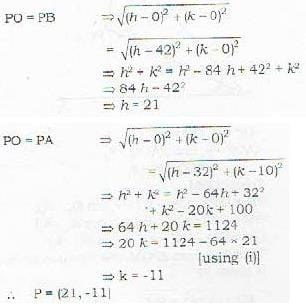Test: Dimensional Geometry - 1 - Question 3

### The points (0,-1), (-2,3), (6,7) , (8,3) from a

Detailed Solution for Test: Dimensional Geometry - 1 - Question 3

Important Rules
R - 1: If the coordinates of three vertices of a triangle are given then it represents a Right -angled Triangle if the product of the slopes of two adjacent sides is-1.
OR
The square of the largest side equals the sum of the squares of the other two sides.
R - 2: If the coordinates of four vertices of a quadrilateral are known, then it represents a
(i)    Rectangle : If

• opposite sides are equal
• diagonals are equal

(ii)    Square : if

• all sides are equal
• angles are right angles

(iii)    Parallelogram : if any one of the following conditions is true

• opposite sides are equal
• opposite sides are parallel
• middle points of the two diagonals coincide

(iv) Rhombus : if

• adjacent sides are not mutually perpendicular
• opposite sides are parallel Now the solution of the Problem

Proof: Let A(0, -1), B(-2, 3), C(6, 7) and D(8, 3) be the given point. Then using the distance formula, we get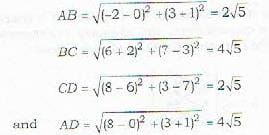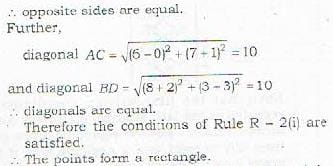Test: Dimensional Geometry - 1 - Question 4

If (x,y) is a point equidiantant from (3,4) and (1,-2), then

Detailed Solution for Test: Dimensional Geometry - 1 - Question 4

Proof:It is given that the point P(x, y) is equidistant from the points A(3,4)and B(1,-2).
i.e PA-PB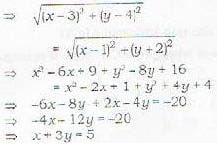Test: Dimensional Geometry - 1 - Question 5

The coordinates of point P(x, y) dividing the line segment joining two points A(x1 , y1) and B(x2, y2)  joining the ratio m: n internally arc given by

Test: Dimensional Geometry - 1 - Question 6

The coordinates of a point P(x, y) which divides the line segment joining A(x1, y1) and B(x2, y2) externally in the ratio m: n, are given by

Test: Dimensional Geometry - 1 - Question 7

The centroid of a triangle is

Detailed Solution for Test: Dimensional Geometry - 1 - Question 7

1. It is  the point of intersection of the median of the triangle.
2. It divides each median in the ratio 2:1.
3. It coordinates are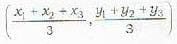Test: Dimensional Geometry - 1 - Question 8

The centroid of the triangle, whose vertices are (3, -5), (-7, 4), (10,-2), is given by

Detailed Solution for Test: Dimensional Geometry - 1 - Question 8

The coordinates of the centroid will be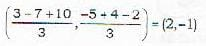Test: Dimensional Geometry - 1 - Question 9

The point dividing the join of (2, 1) and (3, 5) externally in the ratio 2 : 3 is given by

Test: Dimensional Geometry - 1 - Question 10

The area of a triangle with vertices as (x1, y1), (x2, y2) and (x3, y3) is given

Test: Dimensional Geometry - 1 - Question 11

The Three coordinate planes divide the whole space into how many octants?

Detailed Solution for Test: Dimensional Geometry - 1 - Question 11

The three coordinate planes divide the whole space into Eight Parts, called octants. These eight octants and signs of the co-ordinates of a point P in each one of them are shown below :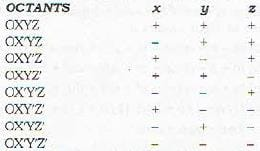Remark : Three fixed lines X'OX Y' O Y and Z' O Z are called x, y and z-axis respectively and their common point of intersection ‘O' is called the origin or pole of coordinates.

Test: Dimensional Geometry - 1 - Question 12

The perpendicular distance of any point P(x,y, z) from the z-axis is given by

Detailed Solution for Test: Dimensional Geometry - 1 - Question 12

We have
1. Distance between two points (x1, y1, z1) and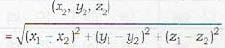2. Distance of a point (x1, y1, z1) from the origin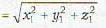3. Distance of a point (x1, y1, z1) from x-axis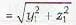4. Distance of a point (x1, y1, z1) from y-axis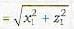5. Distance of a point (x1, y1, z1) from z-axis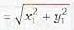Test: Dimensional Geometry - 1 - Question 13

What is the locus of a point for which y=b?

Detailed Solution for Test: Dimensional Geometry - 1 - Question 13

Note: We have

1. Plane | | to xy piane at a distance c, z = c
2. Plane; | | to t/x plane ai a distance a, x = a
3. Plane | to xx plane at a distance b ,y = b

Remark:- In particular, the equations of YZ, ZX and XY planes are x = 0, y = 0 and z = 0 respectively.

Test: Dimensional Geometry - 1 - Question 14

What is the locus of a point for which x = a and y= b?

Detailed Solution for Test: Dimensional Geometry - 1 - Question 14

x = a is a plane | | to YZ plane
y = b is a plane | | to ZX plane
Therefore x = a and y = b is a line parallel to z-axis
Note : Remember that two planes intersect in a line

Test: Dimensional Geometry - 1 - Question 15

What are the length of the edges of the rectangular parallolopiped formed by planes drawn through the point (a. b, c) and (p, q, r) parallel to the coordinate planes?

Detailed Solution for Test: Dimensional Geometry - 1 - Question 15

Lengths of the edges will be
p - a, q - b and r - c

Test: Dimensional Geometry - 1 - Question 16

Which of the following figures is formed by the points whose coordinates are (0,7,10), (-1,6,6), (-4,9,6)?

Detailed Solution for Test: Dimensional Geometry - 1 - Question 16

The distance between two points in a three dimensional can be calculated as :-

d = [(x2-x1)2 + (y2-y1)2 + (z2-z1)2]½

The coordinates are P(0,7,10), Q(-1,6,6), R(-4,9,6)

PQ = [(0+1)2 + (7-6)2 + (10-6)^2]½

PQ = [1+1+16]½

PQ = ½

QR = [(-1-4)2 + (6-9)2 + (6-6)^2]½

QR = [9+9+0]

QR = ½

PR = [(0+4)2 + (7-9)2 + (10-6)^2]½

PR = [16 + 4 + 16]½

PR = 6

As we can see that (PR)2 = (QR)2 + (PQ)2

I.e. isoceles and right triangle

Test: Dimensional Geometry - 1 - Question 17

The point (4,3,-6) lies in which of the eight octants? (x',y',z', are negative version of x,y,z)

Test: Dimensional Geometry - 1 - Question 18

What is radius of a sphere passing through the four (3,2,2), (-1,1,3), (0,5,6), (2,1,2) and having centre (1,3,4)?

Detailed Solution for Test: Dimensional Geometry - 1 - Question 18

Centre o f the sphere = ( 1 , 3 , 4 )
Sphere passes through (3. 2, 2)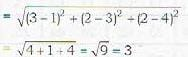Remark: Sphere passes through four points.
Note: Distance of any one of the ratio m : n arc given as follows

Test: Dimensional Geometry - 1 - Question 19

The YZ plane divides the line joining the points (2, 4. 5), (3, 5, -4) in the ratio

Detailed Solution for Test: Dimensional Geometry - 1 - Question 19

The coordinates (x, y, z) of a point P which divides the line joining A(x1, y1 z1 ) and B(x2, y2, z2) in the ratio m : n are given as follows:
1. P divides AB internally in the ratio m : n
Then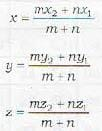2. P divides AB externally in the ratio m : n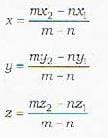Now yz plane is given by x = 0. It means that suppose the point whose x-coordinate is zero divides the line joining (2, 4, 5) and (3. 5, -6) in the ratio m : n.
Then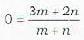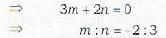Test: Dimensional Geometry - 1 - Question 20

What are the coordinates of the centroid of the triangle whose vertices are (3, 2, 0), (5, 3, 2} and (-9, 6, -3)?

Detailed Solution for Test: Dimensional Geometry - 1 - Question 20

Centroid of the triangle whose vertices are (x1, y1, z1), (x2, y2, z2), (x3, y3, z3) are given by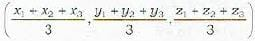In this question, the vertices of the triangle are (3, 2, 0), (5, 3. 2) and (-9, 6, -3).
∴The coordinates of the centroid are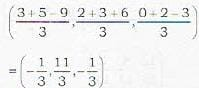1. Tetrahedron is a pyramid with a triangular base.
2. It has four vertices.
3. It has four faces. If BCD is the triangular base and A the top ertex, then the four faces are BCD, ABC, ACDand ABD
4. It has six edges, AB, AC, AD, BC, CD and DB are its six edges.
5. If (x1,y1,z1) , (x2,y2,z2), (x3,y3,z3) and (x4,y4,z4) are the coordinates of the vertices, then the Coordinates of its centroid (centre of gravity) are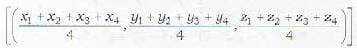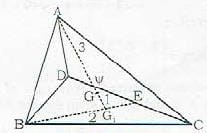above figure shows a tetrahedron. Rase triangle is BCD. The centre of gravity G of the tetrahedron divides the line AG. in the ratio 3:1, where G, is the centre of gravity of the base triangle BC (E is the middle point of DC and Gt divides BE in :he ratio 2 : 1).
6. The volume V of the tetrahedron is given by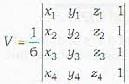## Topic-wise Tests & Solved Examples for IIT JAM Mathematics

27 docs|150 tests
 Use Code STAYHOME200 and get INR 200 additional OFF Use Coupon Code
Information about Test: Dimensional Geometry - 1 Page
In this test you can find the Exam questions for Test: Dimensional Geometry - 1 solved & explained in the simplest way possible. Besides giving Questions and answers for Test: Dimensional Geometry - 1, EduRev gives you an ample number of Online tests for practice

## Topic-wise Tests & Solved Examples for IIT JAM Mathematics

27 docs|150 tests